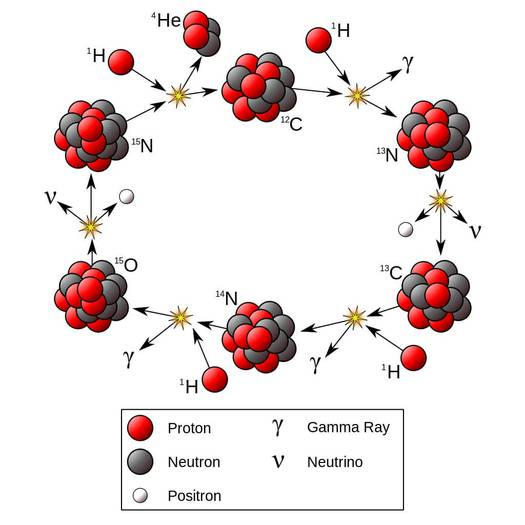# CNO CycleAlmost all star radiates energy by the process of two fusion reaction types; CNO ( carbon-nitrogen–oxygen) and p-p (proton-proton) chain cycle. The fusion reaction types in stars depend on the mass of stars. Hypothetically, the CNO cycle is dominant in stars that are more than 1.3 times the solar mass and p-p chain reaction cycle is dominant in stars having mass less than or equal to that of the solar mass. [1 solar mass ≈ 2✕1030 kg]

In the CNO cycle, four hydrogen atoms are captured by Carbon and Nitrogen atom in different steps and again regenerated carbon atom at last. Then the cycle repeats.

In details;

In the first step, a carbon-12 (12C) nucleus captures a hydrogen nucleus (1H, a proton) to form a nucleus of nitrogen-13 (13N). A gamma-ray (𝛄) is emitted in this process.

12C + 1H      →     13N + 𝛄 + 1.95 MeV

In the second step, the nitrogen-13 nucleus is unstable, so decompose and emits a positive electron (positron, e+) and becomes carbon-13 (13C).

13N         →        13C + e+ + ve+ 1.20 MeV

(vis electron neutrino).

In the third step, the 13C nucleus then captures another proton and becomes nitrogen-14 (14N), and emits another gamma-ray.

13C + 1H       →      14N + 𝛄 + 7.54 MeV

In the fourth step, the nitrogen-14 (14N) from the third step captures a proton to form oxygen-15 (15O) and results in the oxygen-15 (15O) by emitting a gamma-ray and energy of 7.35 MeV.

14N + 1H        →      15O + 𝛄 + 7.35 MeV

Now in the fifth steps, Oxygen-15 becomes nitrogen-15 via beta decay (captures a fast-moving proton).

15O        →       15N + e+ + ve + 1.73 MeV

In the final step, Nitrogen-15 captures a proton and produces a helium nucleus (alpha particle) and carbon-12, and the similar reaction cycle is commenced.

15N + 1H       →      12C + 4He + 4.96 MeV

Hence, the carbon-12 nucleus used in the initial reaction is regenerated in the final one and hence acts as a catalyst for the whole cycle and the total energy released in one cycle is 26.73 MeV (when the two positrons emitted annihilate with two ambient electrons there produces an additional 2.04 MeV).